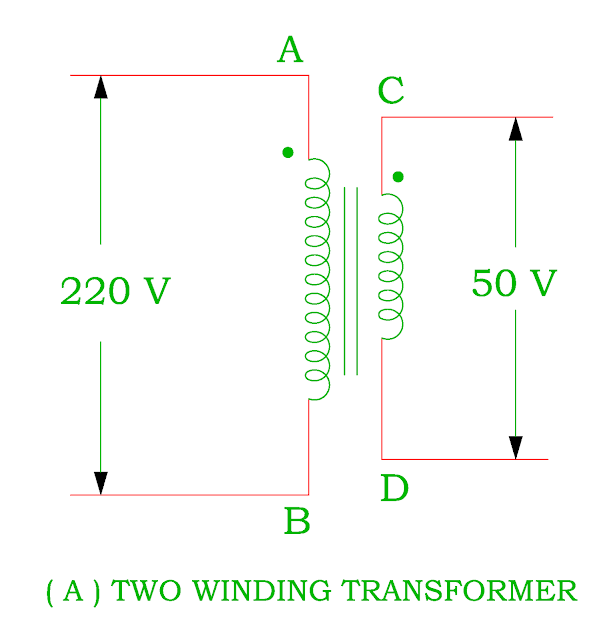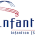## 03/11/2017

### Working of Auto transformer, Saving in Copper Material

• It consists of only single winding which is common to both primary and secondary winding
• It has two end terminals ( PR) acts as primary and one end terminal ( either P or R ) and inter mediate terminal Q acts as a secondary winding ( Figure A ).
• The position of the intermediate terminal Q determines whether auto – transformer works as step down transformer or step up transformer.
• The theory and operation of the auto - transformer is similar to that of conventional two winding transformer
• The auto transformer is cheaper than the ordinary transformer because of single winding uses less copper than the two winding.
• Since the voltage / turn is equal in both winding, the voltage develops across each winding depends on number of turns.• Figure B shows a step down transformer in which PQ winding acts as a primary having N1 turns and QR winding acts as a secondary winding consists of N2 turns.
• If the supply voltage V1 is given to primary winding

Induced emf per turn ( Et ) = V1 / N1
As we know that the value of Et is same for both winding

Secondary voltage V2 = Et × N2

Saving of Copper in Auto transformer as step down transformer
• The weight of winding material ( Copper ) is proportional to the length and area of cross section of the conductors.

W a Volume of material
W a ( a × L )
• The cross section of the conductor depends on current ( I ) and length of conductors is proportional to the number of turns ( N ).
• Therefore the weight of copper is directly proportional to ampere - turns ( NI ).

Weight of the copper in the ordinary transformer ( WO ) = N1I1 + N2I2
Weight of copper in the auto transformer ( Wa ) =
Weight of copper in part PQ a I1 ( N1 – N2 )
Weight of copper in part QR a ( I2 – I1 ) N2
Total weight of copper in the auto transformer a I1 ( N1 – N2 ) + ( I2 – I1 )
N2
a ( I1N1 + I2N2 – 2N2I1 )
Weight of copper in auto transformer ( Wa ) / Weight of copper in ordinary transformer ( WO ) =  ( I1N1 + I2N2 – 2N2I1 ) / ( I1N1 + I2N2 )
= ( I1N1 + I1N1 – 2N2I1 ) / 2N1I1  ( As N1I1 = N2I2 )
= ( 2I1N1 / 2I1N1 ) – ( 2N2I1 / 2I1N1 )
= ( 1 ) – ( K )     ( As K = N2 / N1 = I1 / I2 )
= 1 – K
Therefore Wa = ( 1 – K ) WO
Saving in Copper = WO – Wa
= WO – ( 1 – K ) WO
= KWO

• Therefore the saving in copper material depends on the value of K ( Voltage transformation ratio ).
• Higher value of K, more saving in copper material

Saving of Copper in Auto transformer as Step up transformer

Weight of the copper in the ordinary transformer ( WO ) = N1I1 + N2I2
Weight of copper in the auto transformer ( Wa ) =
Weight of copper in part PR a I2 ( N2 – N1 )
Weight of copper in part QR a ( I– I) N1
Total weight of copper in the auto transformer a I2 ( N2 – N1 ) + ( I– I)
N1
a ( I1N1 + I2N2 – 2N1I2 )
Weight of copper in auto transformer ( Wa ) / Weight of copper in ordinary transformer ( WO ) = ( I1N1 + I2N2 – 2N1I2 ) / ( I1N1 + I2N2 )
= ( I1N1 + I1N1 – 2N1I2 ) / 2N1I1  ( As N1I1 = N2I2 )
= ( 2I1N1 / 2I1N1 ) – ( 2N1I2 / 2 N1I1 )
= ( 1 ) – ( 1 / K )     ( As K = N2 / N1 = I1 / I2 )
= ( 1 – 1 / K )
Therefore W= [ ( 1 – 1 / K ) ] WO
Saving in copper = WO – [ ( 1 – 1 / K ) ] WO
= [ 1 – 1 + 1 / K ] WO
= ( 1 / K ) WO
• Therefore the saving in copper material depends on the value of 1 / K.
• Lower value of K, more saving in copper material
Application
• The auto – transformer is used for starting of synchronous motor and induction motor. Its winding is used only during starting period of the motor for a few seconds.
• It is used as a furnace transformer for getting suitable supply voltage for furnace winding from 230 V supply.
• It is used as laboratory equipment to get suitable supply voltage as per requirement.
• To compensate the small voltage drop in a distribution cable by giving small voltage boost.
• It may be used as interconnecting transformer in 132 kV / 66 kV supply system.
Connection of two winding transformer into Auto – transformer
• The winding connection diagram of an auto transformer from two winding transformer may be as ( I ) Step down transformer ( II ) Step up transformerConversion of Two winding Transformer into Step down Auto Transformer

Conversion of Two winding Transformer into Step up Auto Transformer

You may also like :

#### 9 comments:

1.Closed focus transformers could genuinely show up positively in relationship with shell outline transformer for high voltage control transformer applications.

Transformer winding is one more standard bit of a transformer upgrade, since this is the ensured current bearing conductors contorted inside ring.Winding Machine

2.Really I enjoy your site with effective and useful information. It is included very nice post with a lot of our resources.thanks for share. i enjoy this post. text auto reply

3.4.Leading load cos(phi) pasor plz

5.This is same as transformer vector diagram
https://myelectrical2015.blogspot.com/2017/10/transformer-on-load.html

6.This is such a great resource that you are providing and you give it away for free. I love seeing blog that understand the value of providing a quality resource for free. purple amethyst ring

7.I havent any word to appreciate this post.....Really i am impressed from this post....the person who create this post it was a great human..thanks for shared this with us. garnet engagement ring

8.This is a well crafted article, if perhaps just about all bloggers provided the same worth of content while you, the web will be a far better place. Please keep it up! Amethyst Rings

9.Really interesting blog. I’m thankful for this valuable information. Ogen Infosystem is a leading Website Designing and Development Company in Delhi. Also, get SEO and all types of Digital Marketing Services at an affordable price.
Web Development Company